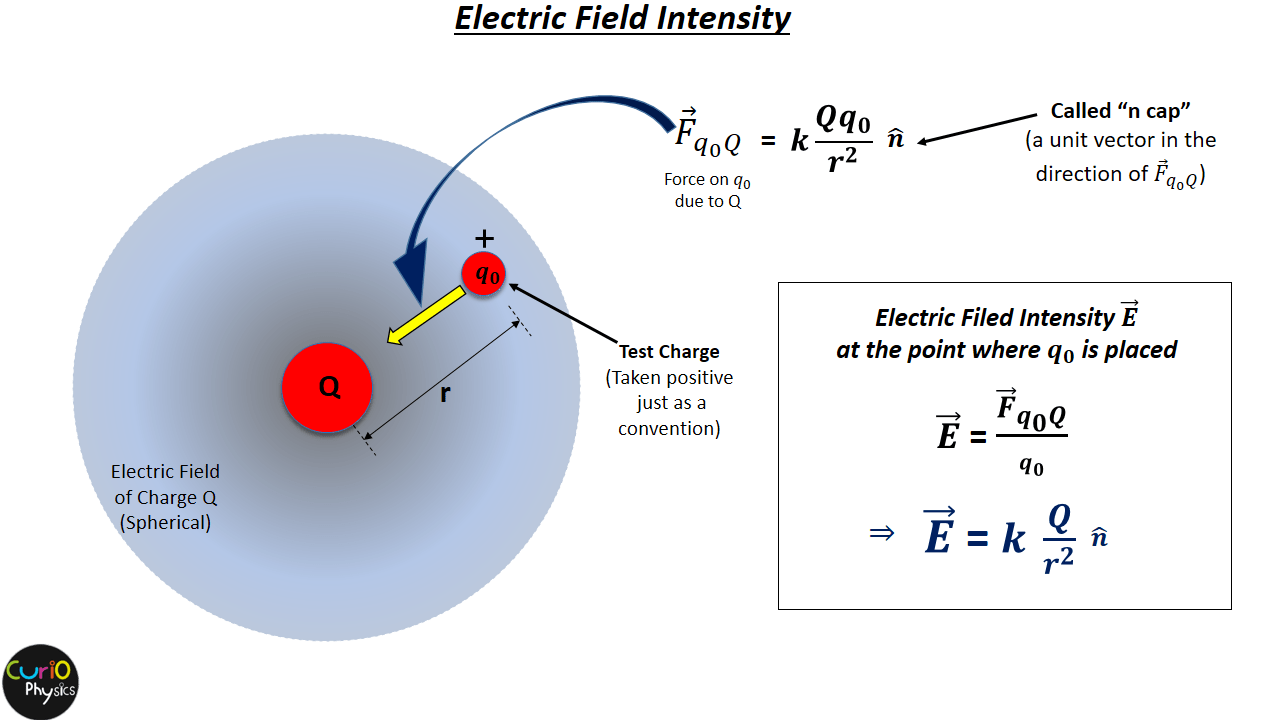# The Electric Field Concept

From Coulomb’s Law,$\displaystyle F=k\frac{{{q}_{1}}{{q}_{2}}}{{{r}^{2}}}$, we conclude that Electric and magnetic forces are :-

• Non-Contact Forces i.e. no physical contact is required for one charge particle to exert a force on another charge particle and they are
• Long-Range Forces i.e. one charge particle exert electric force on another charge particle even if they are at a large distance from each others and
• There is no time(t) dependence of force F

Let us discuss the last point of  no time dependence of F.

For that consider two charge particles A and B. Let us represent the electrostatic force “on B due to A” by$\displaystyle {{\vec{F}}_{BA}}$ . The direction of$\displaystyle {{\vec{F}}_{BA}}$ must always remain from B to A. Now if the charge A starts moving then the direction and magnitude of$\displaystyle {{\vec{F}}_{BA}}$ must also change, i.e. the force vector must pivot to follow A.Now the question here is does this happen instantly? Or is there some time delay between the movement of Charge A and the response of force vector$\displaystyle {{\vec{F}}_{BA}}$.

The question becomes more troubling if we assume the charge particles to be at a very large distance (a few light years away) from each other. Will in that case the response will be instantaneous or there will be some time delay??

On the other hand, looking at Coulomb’s law, there is not any time dependence for the application of electrostatic force, i.e. the electrostatic force acts instantly.

This idea of instantaneous transmission of force between charge particles become inconvincible to most scientists by the beginning of the 19th century.

##### electric field concept by michael faraday

Michael Faraday (22 September 1791 – 25 August 1867) first came up with the idea to call the region around a charge particle as “field”, which is now called as electric field. The word “field”, actually comes from mathematics, which describes a function that assigns a vector to every point in space. According to Faraday long-range forces (electric and gravitational) operates by alternation of space, i.e., a charge particle first alters or modifies the space around it, and then this alternation of space interact with other charge particles.Michael Faraday’s mathematical abilities were limited to simple algebra, but he was an excellent experimentalist and he used to convey his ideas in clear and simple language with his imagination.

Later James Clerk Maxwell took the work of Faraday and others and summarized it in a set of four equations in 1865, which is the basis of all modern theories of electromagnetic phenomena.#### Manoj Kumar Verma

Hi, I'm Manoj Kumar Verma, a physics faculty having 7 years of teaching experience. I have done B.Tech (E.E.). I am also a YouTuber and Blogger. This blog is dedicated to help students learn the physics concepts easily.

View all posts

## 1 Comment

•Kavita says: